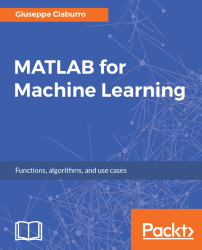•#### MATLAB for Machine Learning#### Overview of this book

MATLAB is the language of choice for many researchers and mathematics experts for machine learning. This book will help you build a foundation in machine learning using MATLAB for beginners. You’ll start by getting your system ready with t he MATLAB environment for machine learning and you’ll see how to easily interact with the Matlab workspace. We’ll then move on to data cleansing, mining and analyzing various data types in machine learning and you’ll see how to display data values on a plot. Next, you’ll get to know about the different types of regression techniques and how to apply them to your data using the MATLAB functions. You’ll understand the basic concepts of neural networks and perform data fitting, pattern recognition, and clustering analysis. Finally, you’ll explore feature selection and extraction techniques for dimensionality reduction for performance improvement. At the end of the book, you will learn to put it all together into real-world cases covering major machine learning algorithms and be comfortable in performing machine learning with MATLAB.
Title PageCreditsForewordwww.PacktPub.comCustomer FeedbackPrefaceFree Chapter
Getting Started with MATLAB Machine LearningImporting and Organizing Data in MATLABFrom Data to Knowledge DiscoveryFinding Relationships between Variables - Regression TechniquesPattern Recognition through Classification AlgorithmsIdentifying Groups of Data Using Clustering MethodsSimulation of Human Thinking - Artificial Neural NetworksImproving the Performance of the Machine Learning Model - Dimensionality ReductionMachine Learning in Practice## Chapter 1. Getting Started with MATLAB Machine Learning

Why is it so difficult for you to accept my orders if you're just a machine? Just a machine? That's like saying that you are just an ape. This is a short dialog between the leading actor and a robot, taken from the movie Automata. In this movie, the robots have two unalterable protocols; the first obliges them to preserve human life and the second limits them from repairing themselves. Why should humans limit the ability of robots to repair themselves? Because robots have a great capacity for self-learning that could lead them to take control of humans, over time maybe.

At least that is what happens in the movie.

But what do we really mean by self-learning? A machine has the ability to learn if it is able to improve its performance through its activities. Therefore, this ability can be used to help humans solve specific problems such as extracting knowledge from large amounts of data. In this chapter, we will be introduced to the basic concepts of machine learning, and then we will take a tour of the different types of algorithm. In addition, an introduction, some background information, and a basic knowledge of the MATLAB environment will be covered. Finally, we will explore the essential tools that MATLAB provides for understanding the amazing world of machine learning.In this chapter, we will cover the following topics:

• Discovering the machine learning capabilities in MATLAB for classification, regression, clustering, and deep learning, including apps for automated model training and code generation
• Taking a tour of the most popular machine learning algorithms to choose the right one for our needs
• Understanding the role of statistics and algebra in machine learning

At the end of the chapter, you will be able to recognize the different machine learning algorithms and the tools that MATLAB provides to handle them.